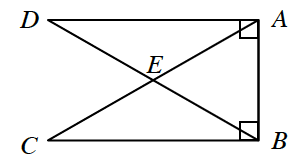### Home > GB8I > Chapter 11 Unit 12 > Lesson INT1: 11.2.3 > Problem11-73

11-73.

In the figure at right, $DE = BE = AE = CE$. Is $ΔDAB ≅ ΔCBA$? Justify your answer using a flowchart.

Make a drawing on paper and mark the four congruent segments.

Both triangles, although they overlap each other, have right angles, and they share a side $AB$.
Review the Math Notes box in section 7.1.7.

$ΔAEB$ is an isosceles triangle, which makes $∠CAB≅∠DBA$.
Consider which triangle congruence condition can be met.